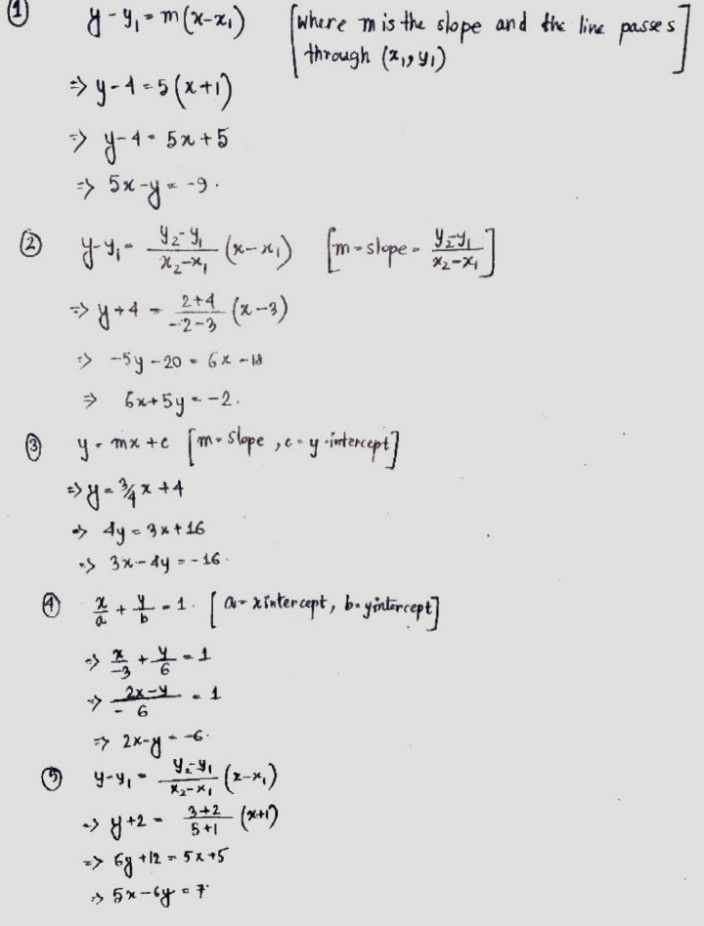Symbol
Problem$a$ In slope intercept $10r1$ $y=t1x+1$ (b) in standard form $ax+by=$ 1. The slope is 5 passing through $1$ 4). The line passes through point $13$ $4\right)$ and $12.2$ 3. The slope is and the $y-tntcrccp$ is $1041$ $1$ The x intercept $-3$ and the $y-lntcrccp$ $106$ $5$ Passing through the points $\left(-1,-2\right)$ and $153$
7th-9th grade
Algebra
Search count: 105
SolutionQanda teacher - missPreetiIf you have any doubts please do let me know dear. if you are satisfied with my answer please do LIKE and give a 5 STAR RATING and coins as THANK U GIFT..! ALL THE BEST..!!!If you have more questions then please ask through preffered match from my profileStudent
Hello! Uhm mam what i need is the Slope intercept form and the standard form. Hope to answer me again. Lovelots